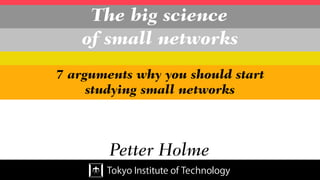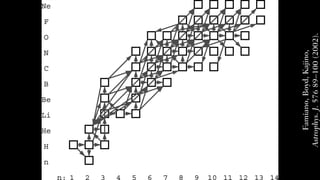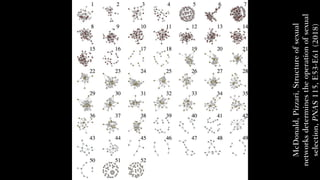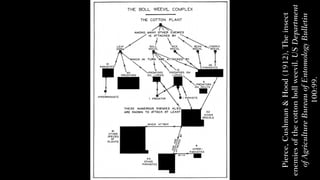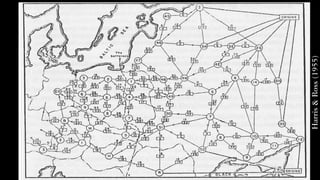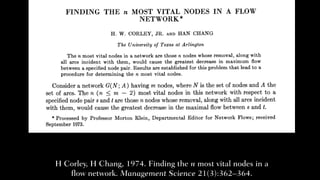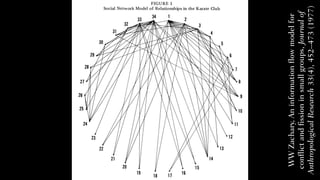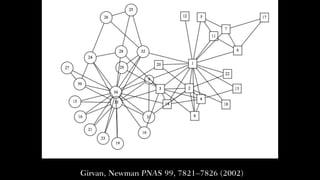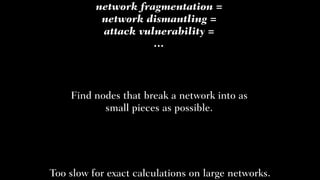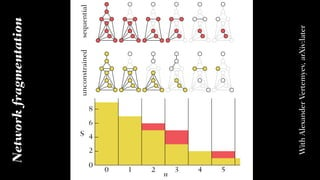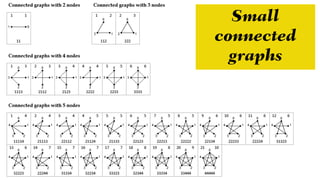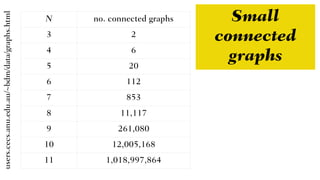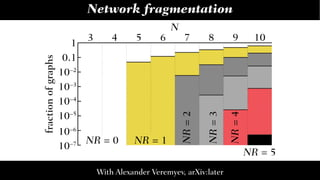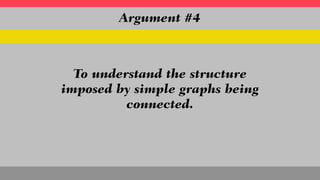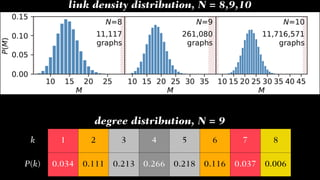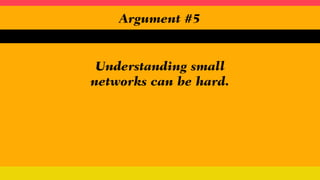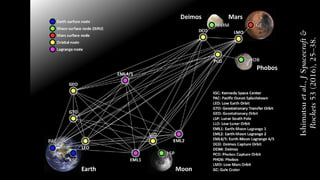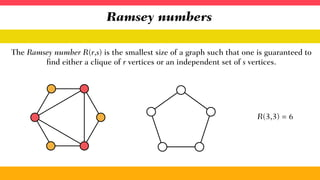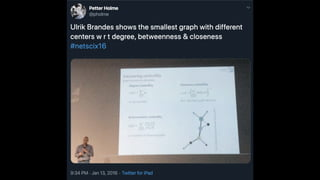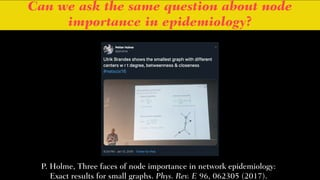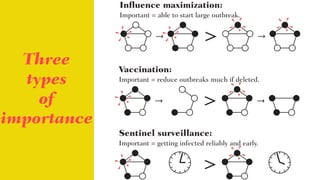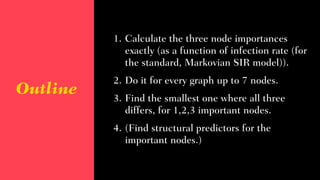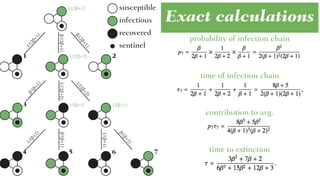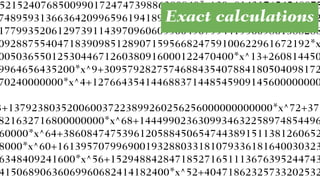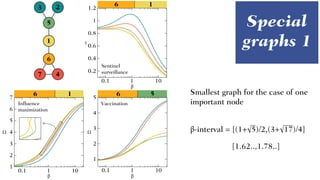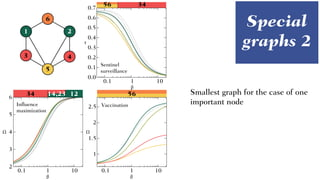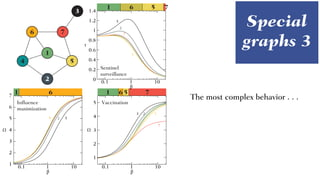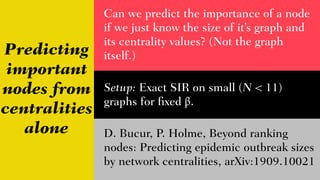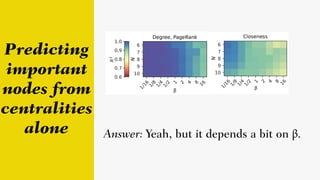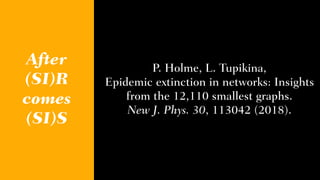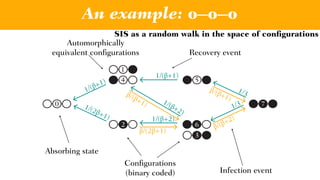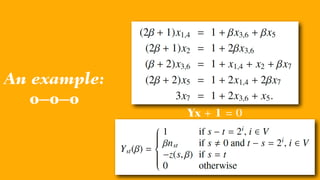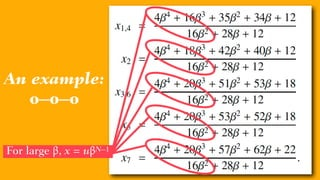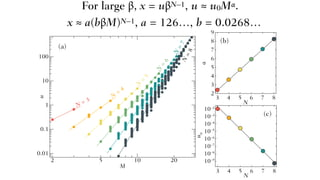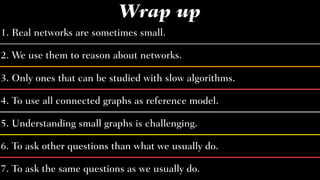1 of 44

### The big science of small networks

1. The big science of small networks Petter Holme 7 arguments why you should start studying small networks
2. Because real networks are sometimes small. Argument #1
3. Famiano,Boyd,Kajino, Astrophys.J.57689–100(2002).
4. McDonald,Pizzari,Structureofsexual networksdeterminestheoperationofsexual selection,PNAS115,E53-E61(2018)
5. Pierce,Cushman&Hood(1912),Theinsect enemiesofthecottonbollweevil.USDepartment ofAgricultureBureauofEntomologyBulletin 100:99.
6. Harris&Ross(1955)
7. H Corley, H Chang, 1974. Finding the n most vital nodes in a flow network. Management Science 21(3):362–364.
8. WWZachary,Aninformationflowmodelfor conflictandfissioninsmallgroups,Journalof AnthropologicalResearch33(4),452–473(1977)
9. Because that’s what we use to reason about networks Argument #2
10. Girvan, Newman PNAS 99, 7821–7826 (2002)
11. To be exact; when exact is slow. Argument #3
12. network fragmentation = network dismantling = attack vulnerability = … Find nodes that break a network into as small pieces as possible. Too slow for exact calculations on large networks.
13. 0 1 3 52 n 4 2 4 6 8 S unconstrainedsequential 0 Networkfragmentation WithAlexanderVertemyev,arXiv:later
14. Small connected graphs
15. N no. connected graphs 3 2 4 6 5 20 6 112 7 853 8 11,117 9 261,080 10 12,005,168 11 1,018,997,864 users.cecs.anu.edu.au/~bdm/data/graphs.html Small connected graphs
16. 1 0.1 10–3 10–4 10–5 10–2 9876543 NR=4 NR=3 NR=2 NR = 1NR = 0 N fractionofgraphs 10–6 10–7 10 NR = 5 Network fragmentation With Alexander Veremyev, arXiv:later
17. To understand the structure imposed by simple graphs being connected. Argument #4
18. k 1 2 3 4 5 6 7 8 P(k) 0.034 0.111 0.213 0.266 0.218 0.116 0.037 0.006 degree distribution, N = 9 link density distribution, N = 8,9,10
19. Understanding small networks can be hard. Argument #5
20. We do these things not because they are easy but because they are hard John F. Kennedy
21. Ishimatsuetal.,JSpacecraft& Rockets53(2016),25–38.
22. Ramsey numbers The Ramsey number R(r,s) is the smallest size of a graph such that one is guaranteed to find either a clique of r vertices or an independent set of s vertices. R(3,3) = 6
23. R(5,5) please. Or we destroy Earth! Mobilize all computers and mathematicians and let’s figure it out.
24. R(6,6) please. Or we destroy Earth! Leave it to the military and hope for the best.
25. To ask other questions than what we usually do. Argument #6
26. Can we ask the same question about node importance in epidemiology? P. Holme, Three faces of node importance in network epidemiology: Exact results for small graphs. Phys. Rev. E 96, 062305 (2017).
27. Three types of importance Influence maximization: Important = able to start large outbreak. Vaccination: Important = reduce outbreaks much if deleted. Sentinel surveillance: Important = getting infected reliably and early.
28. Outline 1. Calculate the three node importances exactly (as a function of infection rate (for the standard, Markovian SIR model)). 2. Do it for every graph up to 7 nodes. 3. Find the smallest one where all three differs, for 1,2,3 important nodes. 4. (Find structural predictors for the important nodes.)
29. susceptible infectious recovered sentinel β/(2β+1) β/(2β+1) 1/(2β+1) β/(β+1) 1/(2β+2) 1/(2β+2) β/(β+1) β/(β+1) 1/(β+1) 1/(β+1) 1/(β+1)1/(β+1) 1/(2β+2) 1/(2β+1) 1 2 3 4 5 6 7 Exact calculations probability of infection chain time of infection chain contribution to avg. time to extinction
30. 5215240768500990172474739886280840*x^29+814217654548875 748959313663642099659619418917821190195217427712*x^25+2 1779935206129739114397096060550049079944195609861388288 09288755404718390985128907159566824759100622961672192*x 0050365501253044671260380916000122470400*x^13+260814450 9964656435200*x^9+3095792827574688435407884180504098172 70240000000*x^4+127664354144688371448545909145600000000 3+137923803520060037223899260256256000000000000*x^72+371 821632716800000000*x^68+1444990236309934632258974854496 60000*x^64+38608474753961205884506547443891511381260652 8000*x^60+161395707996900193288033181079336181640030323 6348409241600*x^56+152948842847185271651113676395244743 41506890636069960682414182400*x^52+40471862325733202532 Exact calculations
31. Special graphs 1 6 6 6 51 12 1 4 5 6 7 3 1 2 3 4 5 6 7 0.1 1 10 0.2 0.4 0.6 0.8 1 1.2 0.1 1 10 1 2 3 4 5 0.1 1 10 β β β Influence maximization Vaccination Sentinel surveillance Ω Ω τ β-interval = [(1+√5)/2,(3+√17)/4] [1.62..,1.78..] Smallest graph for the case of one important node
32. 34 14,23 12 56 3456 21 3 6 5 4 Influence maximization 3 4 5 0.1 1 10 1 1.5 2 2.5 0.1 1 10 0.1 0.2 0.3 0.4 0.5 0.6 0.1 1 10 0.0 0.7 2 6 Sentinel surveillance Vaccination β β β Ω Ω τ Special graphs 2 Smallest graph for the case of one important node
33. 7 1 6 75 1 6 751 6 1 2 3 4 5 0.1 1 10 1 2 3 4 5 6 7 0.1 1 10 0 0.2 0.4 0.6 0.8 1 1.2 1.4 0.1 1 10 326 3 2 5 3 2 7 5 Sentinel surveillance VaccinationInfluence maximization Ω Ω τ 2 1 4 5 6 7 3 β β β Special graphs 3 The most complex behavior . . .
34. Predicting important nodes from centralities alone Can we predict the importance of a node if we just know the size of it’s graph and its centrality values? (Not the graph itself.) D. Bucur, P. Holme, Beyond ranking nodes: Predicting epidemic outbreak sizes by network centralities, arXiv:1909.10021 Setup: Exact SIR on small (N < 11) graphs for fixed β.
35. Predicting important nodes from centralities alone Answer: Yeah, but it depends a bit on β.
36. P. Holme, L. Tupikina, Epidemic extinction in networks: Insights from the 12,110 smallest graphs. New J. Phys. 30, 113042 (2018). After (SI)R comes (SI)S
37. To ask the same questions as we usually do. Argument #7
38. 1/(2β+1) 1/(β+1) 1/(β+2) 1/(β+1) 1/(β+2) 1/3 1/3 β/(β+2) β/(β+1) β/(β+1) β/(2β+1) 0 4 1 2 5 6 3 7 An example: o–o–o Absorbing state Automorphically equivalent configurations Recovery event Infection event SIS as a random walk in the space of configurations Configurations (binary coded)
39. Yx + 1 = 0 An example: o–o–o
40. An example: o–o–o For large β, x = uβN–1
41. N = 3 N = 4 N = 5 N = 6 N = 7N =8 0.01 0.1 1 10 100 105 202 2 3 4 5 6 7 8 9 3 4 5 6 7 8 3 4 5 6 7 8 M u N N 10–2 10–4 10–6 10–8 10–9 10–7 10–5 10–3 u0 α (a) (b) (c) For large β, x = uβN–1, u ≈ u0Mα. x ≈ a(bβM)N–1, a = 126…, b = 0.0268…
42. 1. Real networks are sometimes small. 2. We use them to reason about networks. 3. Only ones that can be studied with slow algorithms. 4. To use all connected graphs as reference model. 6. To ask other questions than what we usually do. 7. To ask the same questions as we usually do. 5. Understanding small graphs is challenging. Wrap up
43. Thank you! Doina Bucur U Twente Liubov Tupikina CRI Paris Alexander Veremyev U Central Florida Funding: Tokyo Tech WRHI JSPS Sumitomo Foundation Homepage: petterhol.me Collaborators: